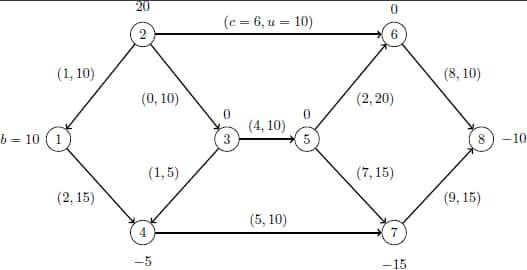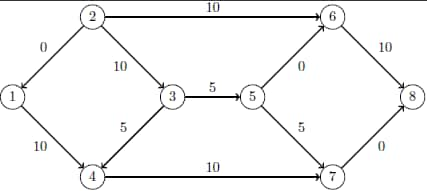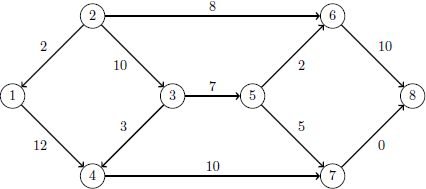### Example 6.5 Using the Network Simplex Solver

This example demonstrates how you can use the network simplex solver to find the minimum-cost flow in a directed graph. Consider the directed graph in Output 6.5.1, which appears in Ahuja, Magnanti, and Orlin (1993).

Output 6.5.1: Minimum Cost Network Flow Problem: DataYou can use the following SAS statements to create the input data sets `nodedata` and `arcdata`:

```data nodedata;
input _node_ \$ _sd_;
datalines;
1    10
2    20
3     0
4    -5
5     0
6     0
7   -15
8   -10
;

data arcdata;
input _tail_ \$ _head_ \$ _lo_ _capac_ _cost_;
datalines;
1    4    0    15    2
2    1    0    10    1
2    3    0    10    0
2    6    0    10    6
3    4    0     5    1
3    5    0    10    4
4    7    0    10    5
5    6    0    20    2
5    7    0    15    7
6    8    0    10    8
7    8    0    15    9
;

```

You can use the following call to PROC OPTMODEL to find the minimum-cost flow:

```proc optmodel;
set <str> NODES;
num supply_demand {NODES};

set <str,str> ARCS;
num arcLower  {ARCS};
num arcUpper  {ARCS};
num arcCost   {ARCS};

arcLower=_lo_ arcUpper=_capac_ arcCost=_cost_;
read data nodedata into NODES=[_node_] supply_demand=_sd_;

var flow {<i,j> in ARCS} >= arcLower[i,j] <= arcUpper[i,j];
min obj = sum {<i,j> in ARCS} arcCost[i,j] * flow[i,j];
con balance {i in NODES}:
sum {<(i),j> in ARCS} flow[i,j] - sum {<j,(i)> in ARCS} flow[j,i]
= supply_demand[i];
solve with lp / algorithm=ns scale=none logfreq=1;
print flow;
quit;
%put &_OROPTMODEL_;

```

The optimal solution is displayed in Output 6.5.2.

Output 6.5.2: Network Simplex Solver: Primal Solution Output

The OPTMODEL Procedure

Problem Summary
Objective Sense Minimization
Objective Function obj
Objective Type Linear

Number of Variables 11
Bounded Above 0
Bounded Below 0
Bounded Below and Above 11
Free 0
Fixed 0

Number of Constraints 8
Linear LE (<=) 0
Linear EQ (=) 8
Linear GE (>=) 0
Linear Range 0

Constraint Coefficients 22

Performance Information
Execution Mode Single-Machine
Number of Threads 1

Solution Summary
Solver LP
Algorithm Network Simplex
Objective Function obj
Solution Status Optimal
Objective Value 270

Primal Infeasibility 0
Dual Infeasibility 0
Bound Infeasibility 0

Iterations 8
Iterations2 0
Presolve Time 0.00
Solution Time 0.00

  flow
1 4 10
2 1 0
2 3 10
2 6 10
3 4 5
3 5 5
4 7 10
5 6 0
5 7 5
6 8 10
7 8 0

The optimal solution is represented graphically in Output 6.5.3.

Output 6.5.3: Minimum Cost Network Flow Problem: Optimal SolutionThe iteration log is displayed in Output 6.5.4.

Output 6.5.4: Log: Solution Progress

 NOTE: There were 11 observations read from the data set WORK.ARCDATA. NOTE: There were 8 observations read from the data set WORK.NODEDATA. NOTE: Problem generation will use 4 threads. NOTE: The problem has 11 variables (0 free, 0 fixed). NOTE: The problem has 8 linear constraints (0 LE, 8 EQ, 0 GE, 0 range). NOTE: The problem has 22 linear constraint coefficients. NOTE: The problem has 0 nonlinear constraints (0 LE, 0 EQ, 0 GE, 0 range). NOTE: The problem is a pure network instance; PRESOLVER=NONE is used. NOTE: The LP presolver value NONE is applied. NOTE: The LP solver is called. NOTE: The Network Simplex algorithm is used. NOTE: The network has 8 rows (100.00%), 11 columns (100.00%), and 1 component. NOTE: The network extraction and setup time is 0.00 seconds. Primal         Primal           Dual Iteration      Objective  Infeasibility  Infeasibility     Time 1              0     20.0000000     89.0000000     0.00 2              0     20.0000000     89.0000000     0.00 3      5.0000000     15.0000000     84.0000000     0.00 4      5.0000000     15.0000000     83.0000000     0.00 5     75.0000000     15.0000000     83.0000000     0.00 6     75.0000000     15.0000000     79.0000000     0.00 7    130.0000000     10.0000000     76.0000000     0.00 8    270.0000000              0              0     0.00 NOTE: The Network Simplex solve time is 0.00 seconds. NOTE: The total Network Simplex solve time is 0.00 seconds. NOTE: Optimal. NOTE: Objective = 270. STATUS=OK ALGORITHM=NS SOLUTION_STATUS=OPTIMAL OBJECTIVE=270 PRIMAL_INFEASIBILITY=0 DUAL_INFEASIBILITY=0 BOUND_INFEASIBILITY=0 ITERATIONS=8 ITERATIONS2=0 PRESOLVE_TIME=0.00 SOLUTION_TIME=0.00

Now, suppose there is a budget on the flow that comes out of arc 2: the total arc cost of flow that comes out of arc 2 cannot exceed 50. You can use the following call to PROC OPTMODEL to find the minimum-cost flow:

```proc optmodel;
set <str> NODES;
num supply_demand {NODES};

set <str,str> ARCS;
num arcLower  {ARCS};
num arcUpper  {ARCS};
num arcCost   {ARCS};

arcLower=_lo_ arcUpper=_capac_ arcCost=_cost_;
read data nodedata into NODES=[_node_] supply_demand=_sd_;

var flow {<i,j> in ARCS} >= arcLower[i,j] <= arcUpper[i,j];
min obj = sum {<i,j> in ARCS} arcCost[i,j] * flow[i,j];
con balance {i in NODES}:
sum {<(i),j> in ARCS} flow[i,j] - sum {<j,(i)> in ARCS} flow[j,i]
= supply_demand[i];
con budgetOn2:
sum {<i,j> in ARCS: i='2'} arcCost[i,j] * flow[i,j] <= 50;
solve with lp / algorithm=ns scale=none logfreq=1;
print flow;
quit;
%put &_OROPTMODEL_;

```

The optimal solution is displayed in Output 6.5.5.

Output 6.5.5: Network Simplex Solver: Primal Solution Output

The OPTMODEL Procedure

Problem Summary
Objective Sense Minimization
Objective Function obj
Objective Type Linear

Number of Variables 11
Bounded Above 0
Bounded Below 0
Bounded Below and Above 11
Free 0
Fixed 0

Number of Constraints 9
Linear LE (<=) 1
Linear EQ (=) 8
Linear GE (>=) 0
Linear Range 0

Constraint Coefficients 24

Performance Information
Execution Mode Single-Machine
Number of Threads 1

Solution Summary
Solver LP
Algorithm Network Simplex
Objective Function obj
Solution Status Optimal
Objective Value 274

Primal Infeasibility 0
Dual Infeasibility 0
Bound Infeasibility 0

Iterations 7
Iterations2 3
Presolve Time 0.02
Solution Time 0.05

  flow
1 4 12
2 1 2
2 3 10
2 6 8
3 4 3
3 5 7
4 7 10
5 6 2
5 7 5
6 8 10
7 8 0

The optimal solution is represented graphically in Output 6.5.6.

Output 6.5.6: Minimum Cost Network Flow Problem: Optimal Solution (with Budget Constraint)The iteration log is displayed in Output 6.5.7. Note that the network simplex solver extracts a subnetwork in this case.

Output 6.5.7: Log: Solution Progress

 NOTE: There were 11 observations read from the data set WORK.ARCDATA. NOTE: There were 8 observations read from the data set WORK.NODEDATA. NOTE: Problem generation will use 4 threads. NOTE: The problem has 11 variables (0 free, 0 fixed). NOTE: The problem has 9 linear constraints (1 LE, 8 EQ, 0 GE, 0 range). NOTE: The problem has 24 linear constraint coefficients. NOTE: The problem has 0 nonlinear constraints (0 LE, 0 EQ, 0 GE, 0 range). NOTE: The LP presolver value AUTOMATIC is applied. NOTE: The LP presolver removed 4 variables and 4 constraints. NOTE: The LP presolver removed 7 constraint coefficients. NOTE: The presolved problem has 7 variables, 5 constraints, and 17 constraint coefficients. NOTE: The LP solver is called. NOTE: The Network Simplex algorithm is used. NOTE: The network has 4 rows (80.00%), 7 columns (100.00%), and 1 component. NOTE: The network extraction and setup time is 0.00 seconds. Primal         Primal           Dual Iteration      Objective  Infeasibility  Infeasibility     Time 1     80.1500000     10.0600000     55.0000000     0.00 2    105.3000000      5.0300000     54.0000000     0.00 3    105.3000000      5.0300000     54.0000000     0.00 4    135.3000000      0.0300000     49.0000000     0.00 5    135.6300000              0     47.0000000     0.00 6    135.6300000              0              0     0.00 7    270.0000000              0              0     0.00 NOTE: The Network Simplex solve time is 0.00 seconds. NOTE: The total Network Simplex solve time is 0.00 seconds. NOTE: Optimal. NOTE: Objective = 270. NOTE: The Dual Simplex algorithm is used. Objective                Entering           Leaving Phase Iteration        Value         Time     Variable           Variable D 2          1    2.700000E+02         0   flow['5','6'] budgetOn2 (S) D 2          2    2.740000E+02         0 D 2          3    2.740000E+02         0 NOTE: Optimal. NOTE: Objective = 274. NOTE: The Simplex solve time is 0.03 seconds. STATUS=OK ALGORITHM=NS SOLUTION_STATUS=OPTIMAL OBJECTIVE=274 PRIMAL_INFEASIBILITY=0 DUAL_INFEASIBILITY=0 BOUND_INFEASIBILITY=0 ITERATIONS=7 ITERATIONS2=3 PRESOLVE_TIME=0.00 SOLUTION_TIME=0.03